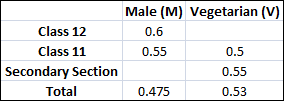### CAT 2007 Question Paper Question 41

Instructions

DIRECTIONS for the following four questions:

The proportion of male students and the proportion of vegetarian students in a school are given below. The school has a total of 800 students, 80% of whom are in the Secondary Section and rest equally divided between Class 11 and 12.Question 41

# In the Secondary Section, 50% of the males are vegetarian.  Which of the following statements is correct?

Solution

x is the fraction of male members in the secondary section.

0.6*80 + 0.55*80 + 640x = 800*0.475

=> 0.6 + 0.55 + 8x = 4.75

=> 8x = 3.6 => x = 0.45

=> 45% are males and 55% are females => 288 males and 352 females

=> There are 144 vegetarian males and 208 vegetarian females .

Also there are 144 non-vegetarian males and 144 non-vegetarian females.

So leaving vegetarian females all others are same in nos.